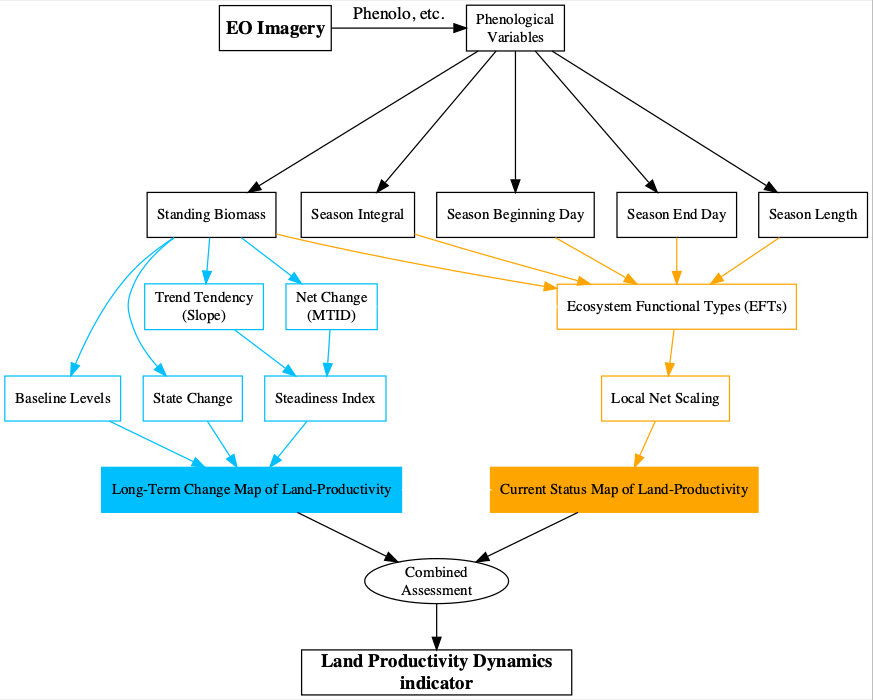# Land Productivity Dynamics: an example to calculate partial LPD indicators with LPDynR

## Introduction

As part of the UN Sustainable Development Goal 15 (Life on Land), the indicator 15.3.1 is adopted to measure the Land Degradation Neutrality (stable —or increasing— state regarding the amount and quality of land resources required to support ecosystem functions and services and enhance food security during a certain period of time). It is a binary indicator (i.e. degraded/not degraded), expressed as the proportion (%) of land that is degraded over total land area, and is based on three sub-indicators: (1) Trends in Land Cover, (2) Land Productivity and (3) Carbon Stocks.

The Land Productivity sub-indicator (LP) refers to the total above-ground Net Primary Production and reflects changes in health and productive capacity of the land. Its declining trends can be usually understood as land degradation. LP is calculated using the Land Productivity Dynamics (LPD) approach, first developed by Ivits and Cherlet (2013). The LPD approach uses phenological and productivity variables derived from time series of remote sensed imagery, particularly the normalized difference vegetation index (NDVI), to estimate ecosystem dynamics and change.

LPD is the methodological basis of the LPDynR package. It is based on a combined assessment of two sources of information, as seen in Figure 1. On the one hand, the first layer is the Long Term Change Map and, in general terms, it shows the tendency of change of land productivity (positive or negative) and the effect that this tendency might have had on a particular original point after a certain period of time. On the other hand, the second layer is the Current Status Map, which provides information on the current efficiency levels of vegetation on the productivity or, in other words, the current level of land productivity in relation to its potential. Further explanations for both branches can be found in Rotllan-Puig, et al. (2021). The final result of the indicator is a categorical map with 5 classes of land productivity dynamics, ranging from declining to increasing productivity.Figure1: Flowchart of the process to calculate the Land Productivity Dynamics indicator and followed by LPDynR

The LPD indicator shows the dynamics of the land productivity giving higher importance to the point at which we are now and where we come from (i.e. the very last year of the time series and the first ones). To better understand the dynamics along the time series as well as the final result, the user might want to calculate several “partial indicators” using only part of the time series in addition to the one for the whole data set. In this vignette we show an example on how to calculate these “partial indicators”.

Note: Please make sure that you have checked the vignette LPD_simple_example included in LPDynR before to go on with this one.

## Example

library(devtools)
install_github("xavi-rp/LPDynR")
library(LPDynR)
library(raster)

Loading a land productivity variable derived from Earth Observation imagery included in LPDynR. A RasterBrick or RasterStack object with time series (each layer is one year).

variables_dir <- paste0(system.file(package='LPDynR'), "/extdata/")   # directory

?sb_cat
sb <- brick(paste0(system.file(package='LPDynR'), "/extdata/sb_cat.tif")) # Standing biomass (integral between the two minimas)

Writing a loop to calculate several “partial LPD indicators” using different parts of the time series. The user can choose the length of these indicators (i.e. number of years) and we suggest to overlap some years of the previous partial indicator with the following. In this example we will calculate partial indicators of 5 years with an overlap of 1.

So first, some parameters for the loop.

ts_length <- 5                                # time series length to run 'partial LPD maps'
ts_years_overlap <- 1                         # number of years of overlapping
wd <- getwd()                                 # user's working directory
partial_dir <- "/LPD_partial"                 # directory to save the 'partial LPD' results in wd
first_year <- 1                               # first year of the whole time series
last_year <- nlayers(sb)                      # last year of the whole time series
last_year_run <- first_year + ts_length - 1   # last year of the 'partial time series'

Then, we can run the loop. This will take some time, be patient!

while(last_year_run <= last_year){

print(paste0("Calculating LPD for years ", first_year, " - ", last_year_run))

# subsetting the years (layers) to run
sb_run <- sb[[first_year:last_year_run]]

# a directory to save the data
if(!dir.exists(partial_dir)) dir.create(partial_dir)
dir2save <- paste0("/", partial_dir, "/LPD_", first_year, "_", last_year_run, "/")
if(!dir.exists(dir2save)) dir.create(dir2save)
setwd(dir2save)

# -----                                        ----- #
#    Here all the steps to calculate the LPD map     #
#       as in the vignette 'LPD_simple_example'      #
# -----                                        ----- #

# Parameters for the loop
first_year <- last_year_run - ts_years_overlap + 1
last_year_run <- first_year + ts_length - 1
setwd(wd)
if(last_year_run > last_year) print("All partial LPD indicators calculated, check the maps!")

}

Finally, you can plot your maps!